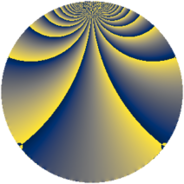# Properties

 Label 700.1.bfLevel $700$ Weight $1$ Character orbit 700.bf Rep. character $\chi_{700}(143,\cdot)$ Character field $\Q(\zeta_{12})$ Dimension $8$ Newform subspaces $1$ Sturm bound $120$ Trace bound $0$

# Related objects

## Defining parameters

 Level: $$N$$ $$=$$ $$700 = 2^{2} \cdot 5^{2} \cdot 7$$ Weight: $$k$$ $$=$$ $$1$$ Character orbit: $$[\chi]$$ $$=$$ 700.bf (of order $$12$$ and degree $$4$$) Character conductor: $$\operatorname{cond}(\chi)$$ $$=$$ $$140$$ Character field: $$\Q(\zeta_{12})$$ Newform subspaces: $$1$$ Sturm bound: $$120$$ Trace bound: $$0$$

## Dimensions

The following table gives the dimensions of various subspaces of $$M_{1}(700, [\chi])$$.

Total New Old
Modular forms 56 24 32
Cusp forms 8 8 0
Eisenstein series 48 16 32

The following table gives the dimensions of subspaces with specified projective image type.

$$D_n$$ $$A_4$$ $$S_4$$ $$A_5$$
Dimension 8 0 0 0

## Trace form

 $$8q + O(q^{10})$$ $$8q + 4q^{16} - 12q^{21} - 16q^{36} - 4q^{46} + 4q^{56} + 12q^{61} + 4q^{81} + 4q^{86} + 12q^{96} + O(q^{100})$$

## Decomposition of $$S_{1}^{\mathrm{new}}(700, [\chi])$$ into newform subspaces

Label Dim. $$A$$ Field Image CM RM Traces $q$-expansion
$$a_2$$ $$a_3$$ $$a_5$$ $$a_7$$
700.1.bf.a $$8$$ $$0.349$$ $$\Q(\zeta_{24})$$ $$D_{6}$$ $$\Q(\sqrt{-5})$$ None $$0$$ $$0$$ $$0$$ $$0$$ $$q-\zeta_{24}^{11}q^{2}+(\zeta_{24}^{5}+\zeta_{24}^{9})q^{3}-\zeta_{24}^{10}q^{4}+\cdots$$